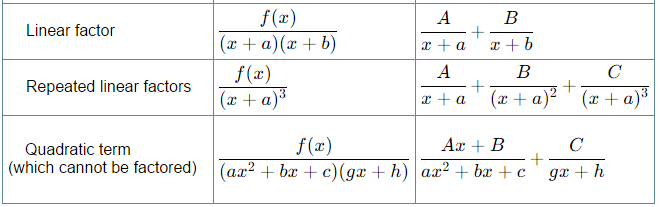# Integration by Partial Fractions

If the integrand (the expression after the integral sign) is in the form of an algebraic fraction and the integral cannot be evaluated by simple methods, the fraction needs to be expressed in partial fractions before integration takes place.

To express a fractional function P(x)/Q(x) as partial fractions, the numerator must be of at least one degree less than the denominator. In other words, the rational function should be proper. Use long division (if necessary) to ensure that the degree of P(x) is less than the degree of Q(x).

Denominator Containing Linear Factors

For each linear factor (ax+b) in the denominator of a rational fraction, there is a partial fraction of the form ​A/(ax+b).

Denominator Containing Repeated Linear Factors

If a linear factor is repeated n times in the denominator, there will be n corresponding partial fractions with degree 1 to n.